• 最小堆C++实现
千次阅读
2020-07-03 22:07:42

最小堆是指在树中，存在一个结点而且该结点有儿子结点，该结点的data域值都小于其儿子结点的data域值，并且它是一个完全二叉树（不是满二叉树）。

这里使用静态数组存储完全二叉树，对于完全二叉树采用顺序存储表示，那么对于任意一个下标为i(1 ≤ i ≤ n)的结点：

1. 父结点为：i / 2（i ≠ 1），若i = 1，则i是根节点。
2. 左子结点：2i（2i ≤ n）， 若不满足则无左子结点。
3. 右子结点：2i + 1(2i + 1 ≤ n)，若不满足则无右子结点。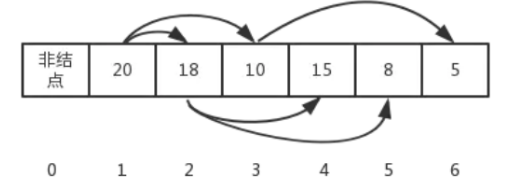## 最小堆的定义：

class MinHeap
{
public:
MinHeap(int size);
~MinHeap();
void Insert(int number);//最小堆插入元素
void Delete();//最小堆删除元素
void swap(int& a, int& b) { int temp = a; a = b; b = temp; }//交换元素
int GetNum() { return count - 1; }//获取最小堆元素个数
void printHeap();//打印堆元素
friend void sort(MinHeap& minheap,int* res,int n);//最小堆排序,res是排序后的数组指针

private:
int* p;//数组指针
int count;//最小堆元素数量
int maxsize;//最小堆的最大元素数量
};

## 最小堆的插入：

最小堆的插入操作可以看成是“结点上浮”。当我们在向最小堆中插入一个结点我们必须满足完全二叉树的标准，那么被插入结点的位置的是固定的。而且要满足父结点关键字值不小于子结点关键字值，那么我们就需要去移动父结点和子结点的相互位置关系。

//最小堆插入元素
void MinHeap::Insert(int number)
{
if (count > maxsize) {
cout << "内存不足，请重新分配空间" << endl;
return;
}
//插入元素
p[count] = number;
//获取当前下标
int curCount = count;
//节点上浮
while (curCount/2)
{
//如果子节点比父节点小，交换节点值，即上浮
if (p[curCount] < p[curCount / 2])
swap(p[curCount], p[curCount / 2]);
//更新当前结点坐标值
curCount /= 2;
}
count++;
}

## 最小堆的删除：

最大堆的删除操作，即从堆的根结点删除元素，同时在将最小堆的最后一个元素移动到根节点处，进行“下降”操作，使其重新平衡成为最小堆。

每次下降操作父节点都需要分对左子节点与右子节点进行判断

//最小堆删除元素
void MinHeap::Delete()
{
int curCount = 1;
int num = GetNum();//获取最小堆元素总数
p[curCount] = p[num];//删除根结点元素，并将其替换为尾部元素
p[num] = 0;
//下降操作
//当子节点存在时
while (2 * curCount <= num - 1)
{
//如果左子节点存在且父节点大于左子节点
if (p[curCount] > p[2 * curCount])
swap(p[curCount], p[2 * curCount]);
//如果右子节点存在且父节点大于右子节点
if(2 * curCount + 1 <= num - 1 && p[curCount] > p[2 * curCount+1])
swap(p[curCount], p[2 * curCount + 1]);
//更新当前结点坐标值
curCount *= 2;
}
count--;
}

## 最小堆的排序：

原理和上面的删除是一样的，你可以认为进行了n次删除就能的到排序结果，不过在删除的根结点的时，把每次得到的根结点值插入到了另外的数组中了，最后返回这个另外的数组

//最小堆排序
void sort(MinHeap& minheap,int res[],int n)
{
//res = new int[minheap.count - 1];
int index = minheap.count;
for (int i = 0; i < n; i++)
{
int curCount = 1;
int num = index - 1;//获取最小堆元素总数
res[i] = minheap.p[curCount];
minheap.p[curCount] = minheap.p[num];//删除根结点元素，并将其替换为尾部元素
//下降操作,当子节点存在时
while (2 * curCount <= num - 1)
{
//如果左子节点存在且父节点大于左子节点
if (minheap.p[curCount] > minheap.p[2 * curCount])
swap(minheap.p[curCount], minheap.p[2 * curCount]);
//如果右子节点存在且父节点大于右子节点
if (2 * curCount + 1 <= num - 1 && minheap.p[curCount] > minheap.p[2 * curCount + 1])
swap(minheap.p[curCount], minheap.p[2 * curCount + 1]);
//更新当前结点坐标值
curCount *= 2;
}
index--;
}
}

## minheap.h

#pragma once
#ifndef MINHEAP_H
#define MINHEAP_H

#include<iostream>
using std::cout;
using std::cin;
using std::endl;

class MinHeap
{
public:
MinHeap(int size);
~MinHeap();
void Insert(int number);//最小堆插入元素
void Delete();//最小堆删除元素
void swap(int& a, int& b) { int temp = a; a = b; b = temp; }//交换元素
int GetNum() { return count - 1; }//获取最小堆元素个数
void printHeap();//打印堆元素
friend void sort(MinHeap& minheap,int* res,int n);//最小堆排序,res是排序后的数组指针

private:
int* p;//数组指针
int count;//最小堆元素数量
int maxsize;//最小堆的最大元素数量
};

MinHeap::MinHeap(int size)
{
maxsize = size;
p = new int[maxsize + 1];
if (p == nullptr) { cout << "内存分配失败！" << endl; exit(-1); }
count = 1;
}

MinHeap::~MinHeap()
{
if (p != nullptr) delete[] p;
}

//最小堆插入元素
void MinHeap::Insert(int number)
{
if (count > maxsize) {
cout << "内存不足，请重新分配空间" << endl;
return;
}
//插入元素
p[count] = number;
//获取当前下标
int curCount = count;
//节点上浮
while (curCount/2)
{
//如果子节点比父节点小，交换节点值，即上浮
if (p[curCount] < p[curCount / 2])
swap(p[curCount], p[curCount / 2]);
//更新当前结点坐标值
curCount /= 2;
}
count++;
}

//最小堆删除元素
void MinHeap::Delete()
{
int curCount = 1;
int num = GetNum();//获取最小堆元素总数
p[curCount] = p[num];//删除根结点元素，并将其替换为尾部元素
p[num] = 0;
//下降操作
//当子节点存在时
while (2 * curCount <= num - 1)
{
//如果左子节点存在且父节点大于左子节点
if (p[curCount] > p[2 * curCount])
swap(p[curCount], p[2 * curCount]);
//如果右子节点存在且父节点大于右子节点
if(2 * curCount + 1 <= num - 1 && p[curCount] > p[2 * curCount+1])
swap(p[curCount], p[2 * curCount + 1]);
//更新当前结点坐标值
curCount *= 2;
}
count--;
}

void MinHeap::printHeap()
{
cout << "开始打印：";
for (int i = 1; i < count; ++i)
cout << p[i] << " ";
cout << endl;
}

//最小堆排序
void sort(MinHeap& minheap,int res[],int n)
{
//res = new int[minheap.count - 1];
int index = minheap.count;
for (int i = 0; i < n; i++)
{
int curCount = 1;
int num = index - 1;//获取最小堆元素总数
res[i] = minheap.p[curCount];
minheap.p[curCount] = minheap.p[num];//删除根结点元素，并将其替换为尾部元素
//下降操作,当子节点存在时
while (2 * curCount <= num - 1)
{
//如果左子节点存在且父节点大于左子节点
if (minheap.p[curCount] > minheap.p[2 * curCount])
swap(minheap.p[curCount], minheap.p[2 * curCount]);
//如果右子节点存在且父节点大于右子节点
if (2 * curCount + 1 <= num - 1 && minheap.p[curCount] > minheap.p[2 * curCount + 1])
swap(minheap.p[curCount], minheap.p[2 * curCount + 1]);
//更新当前结点坐标值
curCount *= 2;
}
index--;
}
}

#endif // !MINHEAP_H



## main.cpp

// MinHeap.cpp : 此文件包含 "main" 函数。程序执行将在此处开始并结束。
//

#include <iostream>
using namespace std;
#include"minheap.h"

int main()
{
MinHeap myheap(256);
int sample[] = { 12,11,23,2,4,5,3,8,9,10 };
for (int i = 0; i < sizeof(sample)/sizeof(int); i++)
myheap.Insert(sample[i]);
cout << "最小堆元素总数："<<myheap.GetNum() << endl;
myheap.printHeap();
myheap.Delete();
cout << "删除根节点！" << endl;
myheap.printHeap();
//for (int i = 0; i < 8; i++)
//	myheap.Delete();
//myheap.printHeap();
int res = { 0 };
sort(myheap, res, 9);
cout << sizeof(res) / sizeof(int) << endl;
for (int i = 0; i < sizeof(res)/sizeof(int); i++)
cout << res[i] << " ";

}数据结构 c++
更多相关内容
• 主要介绍了C语言实现基于最大堆和最小堆的堆排序算法示例,分别是基于最大堆的升序排序和基于最小堆的降序排序实例,需要的朋友可以参考下
• 最小堆的特点是其父节点的值不大于任何一个字节点的值， 实现的是升序排序。 最小堆实现排序的原理，构建一个堆，不断的删除堆顶，这里的删除并不是完全删除， 而是将堆顶移动到末尾，然后父节点开始下沉操作，最后...
• ## 最小堆

千次阅读 2020-10-16 10:45:05
一、 满二叉树 一个深度为k，节点个数为2^k-1的二叉树为满二叉树，即一棵树深度为k，没有空位。...最小堆是一种经过排序的完全二叉树，其中任意非终端节点数值均不大于其左子节点和右子节点的值。 如果一棵..

# 一、 满二叉树

一个深度为k，节点个数为2^k-1的二叉树为满二叉树，即一棵树深度为k，没有空位。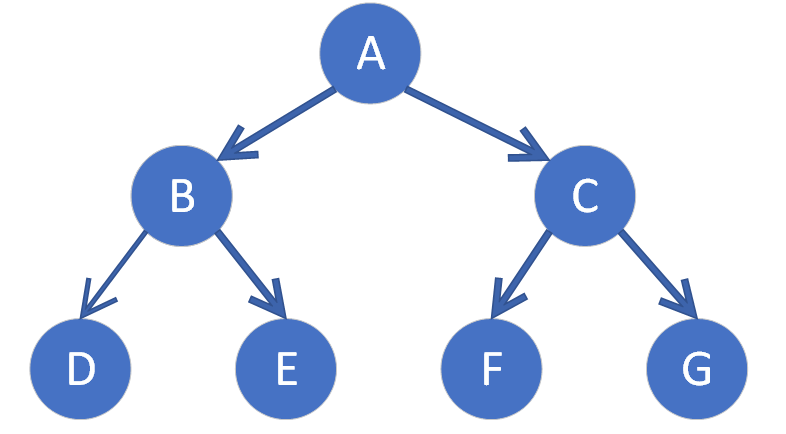# 二、完全二叉树

一棵深度为k有n个节点的二叉树，对树中节点按从上至下、从左至右的顺序进行编号，如果编号为i(1<=i<=n)的节点与满二叉树中编号为i的节点的二叉树中位置相同，则这棵树为完全二叉树。满二叉树是特殊的完全二叉树。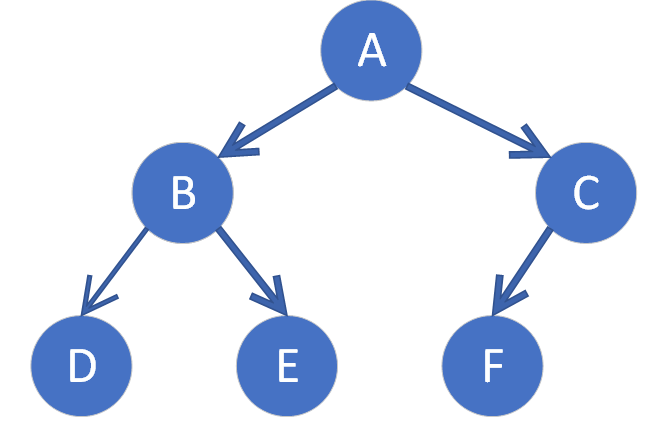# 三、完全二叉树与满二叉树性质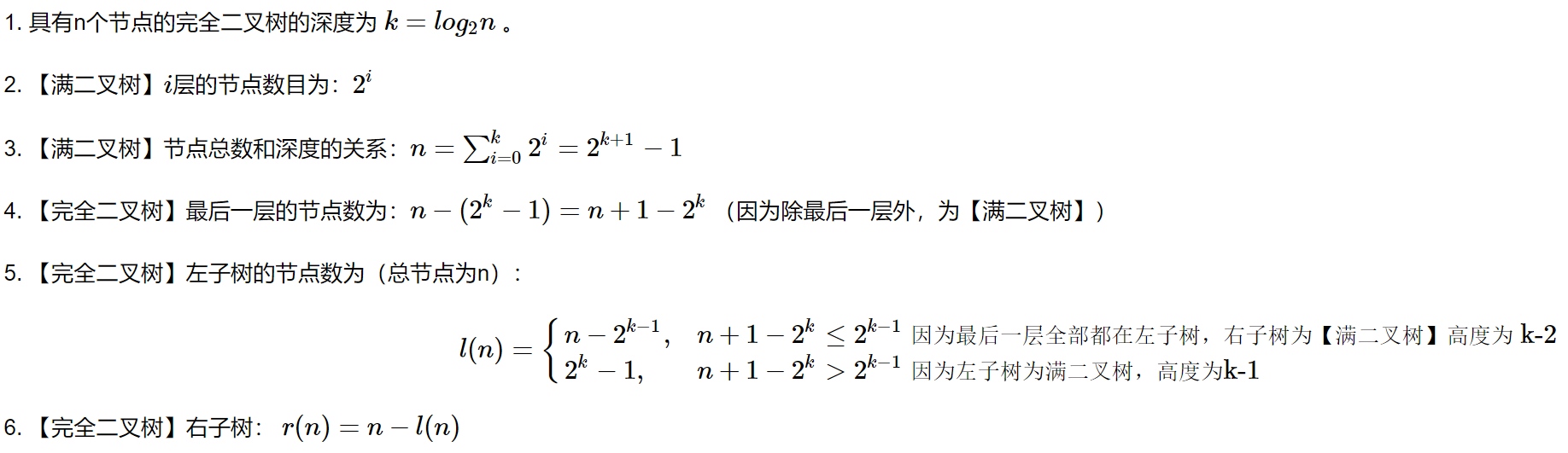# 四、最小堆

最小堆是一种经过排序的完全二叉树，其中任意非终端节点数值均不大于其左子节点和右子节点的值。

如果一棵二叉树满足最小堆的要求，那么，堆顶（根节点）也就是整个序列的最小元素。如果从广度优先的方式从根节点开始遍历，可以构成序列。反过来，可以推演出序列构成二叉树的公式为：

•  对于序列下标为i（下标从0开始）的元素，左孩子的下标为left(i)=i*2+1，右孩子下标为right(i)=left(i)+1。

# 五、二叉树调整为最小堆的方法

1)      倒序遍历数列，因为下标在size/2之后的节点都是叶子结点，所以可以从size/2-1位置开始倒序遍历，减少比较次数。

2)      对二叉树中的元素挨个进行沉降处理，沉降过程为：把遍历到的节点与左右子节点中的最小值比对，如果比最小值要大，那么和孩子节点交换数据，反之则不作处理，继续倒序遍历。

3)      沉降后的节点，再次沉降，直到叶子节点。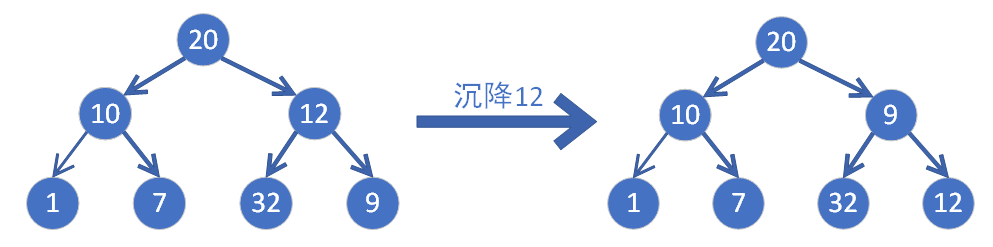# 六、最小堆代码实现

package io.guangsoft;

public class MinPriorityQueue <T extends Comparable> {
//存储堆中元素
private T[] items;
//记录堆中元素个数
private int total;
public MinPriorityQueue(int capacity) {
items = (T[])new Comparable[capacity + 1];
this.total = 0;
}
//获取队列中元素个数
public int size() {
}
//判断队列是否为空
public boolean isEmpty() {
}
//判断堆中索引i处的元素是否小于索引j处的元素
private boolean less(int i, int j) {
return items[i].compareTo(items[j]) < 0;
}
//交换堆中i索引和j索引处的值
private void swap(int i, int j) {
T tmp = items[i];
items[i] = items[j];
items[j] = tmp;
}
//往堆中插入一个元素
public void insert(T t) {
items[++total] = t;
swim(total);
}
//删除堆中最小元素，并返回这个最小元素
public T delMin() {
T min = items;
swap(1, total);
total--;
sink(1);
return min;
}
//使用上浮算法，使索引k处的元素能在堆中处于一个正确的位置
private void swim(int k) {
//通过循环比较当前节点和其父节点的大小
while(k > 1) {
if(less(k, k / 2)) {
swap(k, k / 2);
}
k /= 2;
}
}
//使用下沉算法，使索引k处的元素能在堆中处于一个正确的位置
private void sink(int k) {
//通过循环比较当前节点和其子节点中较小值
while(2 * k <= total) {
//找到子节点中的较小值
int min;
if(2 * k + 1 <= total) {
if(less(2 * k, 2 * k + 1)) {
min = 2 * k;
} else {
min = 2 * k + 1;
}
} else {
min = 2 * k;
}
//判断当前节点和较小值的大小
if(less(k, min)) {
break;
}
swap(k, min);
k = min;
}
}
//主类
public static void main(String args[]) {
//创建最小优先队列
MinPriorityQueue<Integer> queue = new MinPriorityQueue<>(8);
//往队列中存数据
queue.insert(1);
queue.insert(6);
queue.insert(2);
queue.insert(5);
queue.insert(7);
queue.insert(3);
queue.insert(8);
queue.insert(4);
//通过循环获取最小优先队列中的元素
while(!queue.isEmpty()) {
Integer min = queue.delMin();
System.out.print(min + ",");
}
}
}

# 七、 PriorityQueue

PriorityQueue是一个基于优先级的无界队列，优先级队列的元素按照其自然顺序进行排序或者根据构造队列时提供的Comparator进行排序。其存储结构为数组，随着不断向优先级队列添加元素，其容量会自动扩容。

• heapify方法负责把序列转化为最小堆，即所谓的建堆。
• siftDown(k,x)方法用于沉降，根据comparator是否为null决定比较方法。沉降方法把不满足最小堆条件的父节点一路沉到最底部。siftDown的时间复杂度不会超出O(log2n)。
• offer方法用于把数据入队，priorityQueue不允许存放null数据，与ArrayList留有扩容余量不同，当数组长度达到极限时才执行扩容。新添加的节点初始位置是在整个队列的末位，二叉树中，它一定是叶子节点，当它比父节点小时，需要进行上浮。
• grow(minCapacity)方法用于扩容，如果旧容量小于64，则扩容一倍+2，否则扩容1.5倍。扩容后校验容量，容量最大支持最大整型值。
• poll方法用来检索并移除此队列的头，最小堆虽然不能保证数列的顺序，但其堆顶元素始终是最小元素，而priorityQueue也只要求出队的对象优先级最高，当出队即堆顶元素移除后，其左孩子会变为堆顶，需要重新调整堆结构。而移除最小堆的任意叶子节点，最小堆性质不变。
展开全文数据结构 算法 堆排序
• 二叉堆（最小堆）+二项堆+斐波那契堆 根基算法导论C++实现
• 最大堆最小堆 问题的提出 给定k个排好序的序列S1，S2…，Sk，用2路合并算法将这k个序列合并成一个序列。假设所采用的2路合并算法合并2个长度分别为m和n的序列需要m+n-1次比较。试设计一个算法确定合并这个序列的最优...
• 但是，比如我有一个数组，建立一个最小堆，然后每次取出最小堆的顶点。建立最小堆需要额外空间？不深究了，归并排序需要额外空间。堆是完全二叉树，所以可以用数组表示。普通的二叉树需要用链表表示。完全二叉树不...

一点疑惑，堆排序是就地排序，所以空间复杂度是 O(1)。但是，比如我有一个数组，建立一个最小堆，然后每次取出最小堆的顶点。建立最小堆需要额外空间？

不深究了，归并排序需要额外空间。

堆是完全二叉树，所以可以用数组表示。普通的二叉树需要用链表表示。

完全二叉树不等于满二叉树。下图是一个完全二叉树。我们数组下标从0开始。

我们可以得到父节点和子节点之前的坐标公式。

leftChild = 2*parent+1;

rightChild = 2*parent +2;

parent = (child-1)/2;//child 为 leftChild 或 rightChild

插入堆

从书上截取的图片，注意，书上描述的是最大堆，我们代码实现的是最小堆。

插入堆后，保存父节点>子节点的性质。代码算法怎么实现呢？我们需要保持最大堆的性质，插入元素后必然需要移动数组。

下面的方法是先把元素插到数组的末尾，然后比较该元素和父亲元素，判断是否需要交换。重复上述步骤，知道遍历完成或者堆已经是最大堆了。代码

/** * 增加一个新元素，步骤是 1. 先把元素插入到 list 的末尾 2. 比较末尾元素和它的父元素，若小于，交换两者 3. * 重复上述步骤，直到到顶点位置或者子元素大于父元素 4. 不一定要遍历堆所有的元素，达到堆的性质后会提前结束 * * @param array */

int child = data.size() - 1;

int parent = (child - 1) / 2;

// 判断是否到达顶点

while (child > 0) {

// 父元素大于子元素，交换，保持父是小的

if (data.get(parent).compareTo(array) > 0) {

data.set(child, data.get(parent));

data.set(parent, array);

child = parent;

parent = (child - 1) / 2;

} else {

// 已经是最小堆了，无需再比较

break;

}

}

}

上面使用的是插入的方法，充分利用原有最大堆的性质。思路很棒！！

删除顶点元素

删除元素，我自己想的时候也想不到好方法。看书上的才明白，也是利用交换的思路。

删除顶点元素，然后将最后一个元素移动到顶点处。再从上往下遍历，判断顶点元素和它子节点是否满足堆的性质，不满足则交换。重复上述步骤，知道遍历完成或者堆已经是最大堆。

Note 删除和插入元素，不一定需要遍历二叉树的所有层，当已经满足最大堆的性质时候，就可以结束。代码

/** * 删除顶点处的元素，步骤是： 1. 把末尾的元素复制到顶点处 2. 然后比较此时顶点的值和左右子树，保持最小堆的性质 3. * 交换顶点和左右子树较小的值 4. 重复上述步骤，直到已经成了最小堆或者遍历完 5. 注意可能存在左子树存在，右子树不存在情况 6. * 不一定要遍历堆所有的元素，达到堆的性质后会提前结束 * * @return 返回被删除的元素 */

public E removeTop() {

if (data.isEmpty())

return null;

E removed = data.get(0);

// 因为一直交换的是最后的元素，这儿将其保存

E last = data.get(data.size() - 1);

data.set(0, last);

data.remove(data.size() - 1);

int parent = 0;

int leftChild = parent * 2 + 1;

int rightChild = parent * 2 + 2;

while (leftChild <= data.size() - 1) {

int minIndex = leftChild;

// 右子树存在，判断左右子树哪个小，保存坐标

// 如果不存在，那么使用左子树的坐标

// 保存较小元素的坐标，可以省去考虑左右子树都存在，只有左存在的情况

if (rightChild <= data.size() - 1) {

if (data.get(rightChild).compareTo(data.get(leftChild)) < 0) {

minIndex = rightChild;

}

}

if (data.get(minIndex).compareTo(last) < 0) {

data.set(parent, data.get(minIndex));

data.set(minIndex, last);

parent = minIndex;

leftChild = parent * 2 + 1;

rightChild = parent * 2 + 2;

} else {

break; // 已经达到了最小堆的性质

}

}

return removed;

}

注意：代码需要考虑左右子树都存在、只有左子树存在的情景。(只有右子树存在是不可能的)。 那么parent需要和left还是right交换呢？

我本来是用了一大堆if判断。

看书上的很简洁，先设置minIndex = leftChild; (因为左子树是肯定存在的)，然后如果右子树存在的情况下 比较左子树和右子树。如果右子树小，minIndex = rightChild; 否则minIndex不变。然后就可以比较minIndex 和 parent了。

而我以前的方法是 先对左右子树的情况比较。找出较小的树，然后和parent比较。 再考虑左子树的情况，比较左子树和parent。这个方法就很冗余。

利用堆进行排序

先将原来的数据入堆，然后依次取出顶点元素。注意，如果是最大堆，得到的降序。如果是最小堆，得到的是升序。

时间复杂度

堆是有二叉树实现的，对于n个元素，建立二叉树的话，数的深度是log(n)。

对于建立一个二叉树，添加一个元素最多需要log(n)步。所以所以元素添加需要nlog(n)步。

注意如果原来数据是升序的，对于建立一个最小堆是最好情景，对于建立一个最大堆时间是最差情景。

进行对排序，也需要调用n次remove方法，每次remove方法最多需要log(n)步骤。需要的总时间的nlog(n)。

全部代码

/** * 最小堆和堆排序, 最小堆，顶点的元素是最小值， 根据《Java 语言程序设计 进阶篇》 p83 改写， 书上是最大堆. 堆排序 * 将元素都存入最小堆中，从最小堆里面每次取出顶点元素 * * @author tomchen * * @param */

public class MinHeap {

// 测试程序

public static void main(String[] args) {

Random r = new Random(System.currentTimeMillis());

// 测试10次

for (int t = 0; t < 10; t++) {

MinHeap heap = new MinHeap();

int mSize = r.nextInt(15);

Integer[] original = new Integer[mSize];

// 堆的长度和元素都是随机

for (int i = 0; i < mSize; i++) {

original[i] = r.nextInt(100);

}

//copy 数组，调用标准库的方法

Integer[] copy = Arrays.copyOf(original, mSize);

Arrays.sort(copy);

//这儿输出 original 还是乱序的，证明 copy 的排序并无影响

System.out.println("original data: " + Arrays.toString(original));

System.out.println("other sorted data: " + Arrays.toString(copy));

// 调用 heap 排序

heapSort(original);

System.out.println("sorted data: " + Arrays.toString(original));

System.out.println("two sort eqyal : " + Arrays.equals(copy,original));

System.out.println("-----------------------------------------");

}

}

public static void heapSort(E[] array) {

MinHeap heap = new MinHeap();

for (int i = 0; i < array.length; i++) {

}

System.out.println("Debug: heap is " + heap);

for (int i = 0; i < array.length; i++) {

array[i] = heap.removeTop();

}

}

private ArrayList data = new ArrayList();

public MinHeap() {

}

/** * 增加一个新元素，步骤是 1. 先把元素插入到 list 的末尾 2. 比较末尾元素和它的父元素，若小于，交换两者 3. * 重复上述步骤，直到到顶点位置或者子元素大于父元素 4. 不一定要遍历堆所有的元素，达到堆的性质后会提前结束 * * @param array */

int child = data.size() - 1;

int parent = (child - 1) / 2;

// 判断是否到达顶点

while (child > 0) {

// 父元素大于子元素，交换，保持父是小的

if (data.get(parent).compareTo(array) > 0) {

data.set(child, data.get(parent));

data.set(parent, array);

child = parent;

parent = (child - 1) / 2;

} else {

// 已经是最小堆了，无需再比较

break;

}

}

}

/** * 删除顶点处的元素，步骤是： 1. 把末尾的元素复制到顶点处 2. 然后比较此时顶点的值和左右子树，保持最小堆的性质 3. * 交换顶点和左右子树较小的值 4. 重复上述步骤，直到已经成了最小堆或者遍历完 5. 注意可能存在左子树存在，右子树不存在情况 6. * 不一定要遍历堆所有的元素，达到堆的性质后会提前结束 * * @return 返回被删除的元素 */

public E removeTop() {

if (data.isEmpty())

return null;

E removed = data.get(0);

// 因为一直交换的是最后的元素，这儿将其保存

E last = data.get(data.size() - 1);

data.set(0, last);

data.remove(data.size() - 1);

int parent = 0;

int leftChild = parent * 2 + 1;

int rightChild = parent * 2 + 2;

while (leftChild <= data.size() - 1) {

int minIndex = leftChild;

// 右子树存在，判断左右子树哪个小，保存坐标

// 如果不存在，那么使用左子树的坐标

// 保存较小元素的坐标，可以省去考虑左右子树都存在，只有左存在的情况

if (rightChild <= data.size() - 1) {

if (data.get(rightChild).compareTo(data.get(leftChild)) < 0) {

minIndex = rightChild;

}

}

if (data.get(minIndex).compareTo(last) < 0) {

data.set(parent, data.get(minIndex));

data.set(minIndex, last);

parent = minIndex;

leftChild = parent * 2 + 1;

rightChild = parent * 2 + 2;

} else {

break; // 已经达到了最小堆的性质

}

}

return removed;

}

@Override

public String toString() {

return data.toString();

}

}

参考文章

展开全文• 输入文件“input.txt”，路径定义在项目默认：Visual Studio 2010\Projects\Poject1\Poject1 以“Huffman”编码对输入进行压缩编码 输出霍夫曼树的结构 输出编码结果、译码结果
• 利用最小堆编程实现给定权值集合下构造相应霍夫曼树的算法，并解决以下问题： 有一电文共使用五种字符a,b,c,d,e,其出现频率依次为4,7,5,2,9。 (1)构造对应的编码哈夫曼树(要求左子树根结点的权小于等于右子树根结点...
• 最小堆，又称小根堆（小顶堆）是指根节点（亦称为堆顶）的值是堆里所有节点值中的最小者

## 一.引言

前面写到了 最大堆的实现应用，趁热打铁把最小堆的也搞定，最小堆一定意思上和最大堆的实现相同，这不过“大”的概念变成了“小”，不同认知下，可以理解为两个是同一个事情，最小堆常用于大规模数据下寻找 Top-K 小的数字，与之前相似，先明确最小堆及其实现的相关概念：

A. 最小堆，又称小根堆（小顶堆）是指根节点（亦称为小堆顶）的值是堆里所有节点值中的最小者

(1) 每个根节点的值都小于其叶子节点

(2) 最小堆是完全二叉树

B.完全二叉树，一个深度为k，节点个数为 2^k - 1 的二叉树即为完全二叉树

(1) 给定 index 寻找父节点

parent = (index - 1) // 2 = (index - 1) >> 2

(2) 给定 index 寻找子节点

left = 2 * index + 1 = (index << 1) + 1
right = 2 * index + 2 = left + 1

## 二.最小堆实现

### 1.初始化

(1) maxSize 为最小堆的初始化数组长度

(2) count 为当前最小堆中元素的个数

(3) show 函数返回当前数组

(4) isEmpty 判断堆中是否包含元素

# 构造最小堆
class MinHeap():
# 初始化最小堆
def __init__(self, maxSize=None):
self.maxSize = maxSize
self.array = [None] * maxSize
self._count = 0

def length(self):
return self._count

def show(self):
if self._count <= 0:
print('null')
print(self.array[: self._count], end=', ')

def isEmpty(self):
if self._count <= 0:
return True
else:
return False

### 2.添加元素

(1) 将新增加的节点添加至树的最后一位，对应的操作就是将数组的对应位置改为新值，并且 count + 1

(2) shift_up 首先找到当前父节点，如果大于父节点直接退出；小于父节点则交换位置，继续 shift_up 寻找比自己小的父节点，直到小于父节点，退出循环

 [1, 3, 8, 5, 7, 9] -> [1, 3, 6, 5, 7, 9, 8]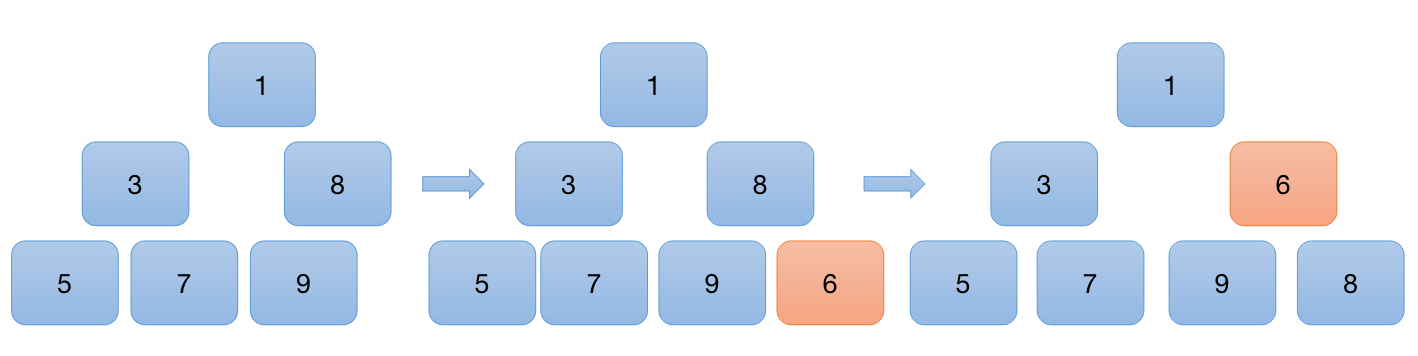def add(self, value):
# 增加元素
if self._count >= self.maxSize:
raise Exception('Full')
self.array[self._count] = value
self._shift_up(self._count)
self._count += 1

def _shift_up(self, index):
# 比较结点与根节点的大小，较小的为根结点
if index > 0:
parent = (index - 1) // 2
if self.array[parent] > self.array[index]:
self.array[parent], self.array[index] = self.array[index], self.array[parent]
self._shift_up(parent)

### 3.弹出堆顶

A.弹出元素

(1) 根据最大堆定义数组第一位即二叉树第一位为最小数值的数字，返回该数字

(2) 将最后一位的值放到第一位准备重建最小堆

(3) 将最后一位元素的值置为 None

B.重建堆

(1) 根据完全二叉树公式获得左右子节点

(2) 比较堆顶元素与左右子节点元素大小，注意判断左右子节点是否存在

(3) 如果当前值非最小，互换当前索引与最小索引，并向下继续递归探索比当前点 smaller 点更小的点

(4) 直到该点小于两个子节点，退出循环

[1, 3, 6, 5, 7, 9, 8] 弹出最小元素1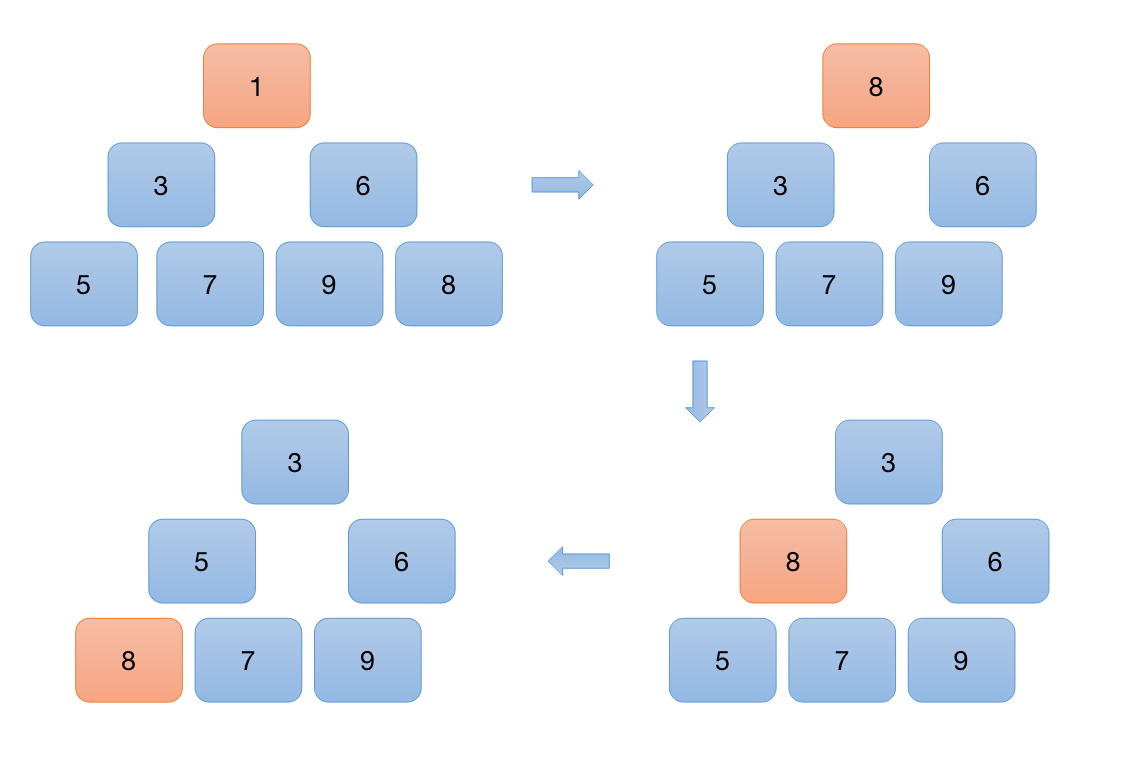def extract(self):
# 获取最小值，并更新数组
if self._count <= 0:
raise Exception('The array is Empty')
value = self.array
self._count -= 1  # 更新数组的长度
self.array = self.array[self._count]  # 将最后一个结点放在前面
self._shift_down(0)

return value

def _shift_down(self, index):
# 此时index 是根结点
if index < self._count:
left = 2 * index + 1
right = 2 * index + 2
# 左右节点都存在
if left < self._count and right < self._count:
left_val = self.array[left]
right_val = self.array[right]
index_val = self.array[index]
# left 最小
if min(left_val, right_val, index_val) == left_val:
self.array[index], self.array[left] = self.array[left], self.array[index]
self._shift_down(left)
# right 最小
if min(left_val, right_val, index_val) == right_val:
self.array[index], self.array[right] = self.array[right], self.array[index]
self._shift_down(right)

# 左叶子结点存在
if left < self._count < right and self.array[left] < self.array[index]:
self.array[left], self.array[index] = self.array[index], self.array[left]
self._shift_down(left)
[1, 3, 7, 6, 5, 9, 8]
3
5
6
7
9
8

这里需要注意有两种情况，一种是左右节点都存在，则需要 left，right，index 三个点同时比大小，也有可能只有左节点（完全二叉树不存在只有右节点情况），此时比较 left，index两个点的大小

### 4.测试

分别添加元素并遍历取出最小值

minHeap = MinHeap(10)

nums = [3, 5, 9, 6, 1, 7, 8]

# 添加元素
for num in nums:

# 弹出最大值
while not minHeap.isEmpty():
print(minHeap.extract())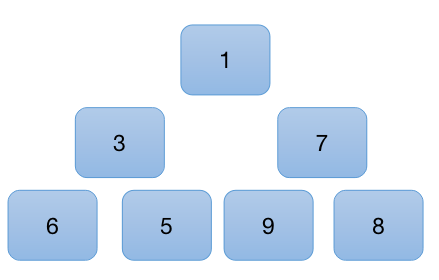[1, 3, 7, 6, 5, 9, 8]
1
3
5
6
7
9
8
展开全文python
• c++ 最小堆 还不错 标准库没有 自己做作业用。
• 一个使用最小堆（通常用于优先级队列）而不是 OrderedDict，第二个使用集合包中的内置 OrderedDict，最后一个使用我自己的 OrderedDict 的简单实现。 观察：所有三个 LRU 缓存都有相同的测试。 我在缓存中运行了...Python
• 本资源是数据结构中利用最小堆实现哈夫曼树的一个C++代码，仅供参考，欢迎指正
•数据结构
• 是特殊的队列，从中取元素是按照元素的优先级大小，而不是元素进入队列的先后顺序。因此，也通常被称为“优先队列”。 的最常用结构是用二叉树表示，不特指的话，他是一棵完全二叉树。因此通常不必用指针，...•c++ 算法
• 最小堆中添加了一个元素后，经过上浮来重新构造最小堆 将一个完全无序的二叉树构造成最小堆，下沉。 package main.java.heap; import java.util.Arrays; /** * 构造最小堆 * * @author Kimizu * @describe...数据结构
• ## 最小堆原理与实现

千次阅读 2020-06-22 13:59:58
基本概念： 1、完全二叉树：若二叉树的深度为h，则除第h层外，其他...最大堆，最小堆类似，以下以最小堆为例进行讲解。 最小堆是满足以下条件的数据结构： 它是一棵完全二叉树； 所有父节点的值小于或等于两个子节点
• 2.最小堆的实现 3.堆排序 0.什么是堆 小堆和大堆分为如下图： 堆需要满足的条件： 1. 必须是二叉树，且必须是完全二叉树 2. 各个父节点必须大于或小于左右结点， 其中最顶层的根结点必须是最大或者最小的 ...python 数据结构与算法
•python
• 堆,优先队列,头文件和队列是同一个#include<queue> #include<iostream> #include<queue> using namespace std; int main() { //最大堆 queue<int>... //最小堆 queue<int算法 c++
• ## Java实现最小堆

千次阅读 2020-08-01 17:01:37
堆，其实是一种优先队列，分为最大堆和最小堆。在最小堆中，它首先是一颗完全二叉树，并且根节点的值，要比左右孩子的值都要小，同时，左右子树也是最小堆。本文包含堆的操作如下： （1）插入一个节点 （2）删除堆...
• 最小堆（小顶堆）是一种二叉树，树中每个节点都小于他的所有子节点，在最小堆的构建和维护过程中最重要的是**上浮(swim)和下沉(sink)**操作。 MinHeap.h #include <algorithm> /* 最小堆类*/ template<...
• 利用最小堆 进行迷宫搜索 class CMinHeap { public: CMinHeap(int nSize) { m_pHeap = new tagPosition[nSize+1]; m_nLast = 0; } void Push(const tagPosition& x) { int i = ++m_nLast; ...
• 个人实现的最小权重的二叉实现，效率很高，适合任意场合下的临时列表排序。 直接执行该文件会执行文件中的测试样例 使用时在头部如此声明 from binaryheap import BinaryHeap bh = BinaryHeap(heap_size) # heap_...
• 最大堆，最小堆类似，以下以最小堆为例进行讲解。 最小堆是满足以下条件的数据结构： 它是一棵完全二叉树 所有父节点的值小于或等于两个子节点的值 1.2 什么是完全二叉树 除了最后一层之外的其他每一层都被完全...TOPK
• 最大堆和最小堆的实现 这一讲讲的还是堆，因此将它归入到堆（一）中。这一篇博客的目的非常简单，就是补充一下，堆的实现代码。Heap是抽象类，它有两个子类，MaxHeap和MinHeap。至于它们的function，我不再赘述了，...• 最小堆和最大堆结构图如下: 堆需要满足的条件： 必须是二叉树，且必须是完全二叉树 各个父节点必须大于或小于左右结点， 其中最顶层的根结点必须是最大或者最小的 实现这样的堆可以采用list或者数组来实现，...python 算法 数据结构 二叉树...